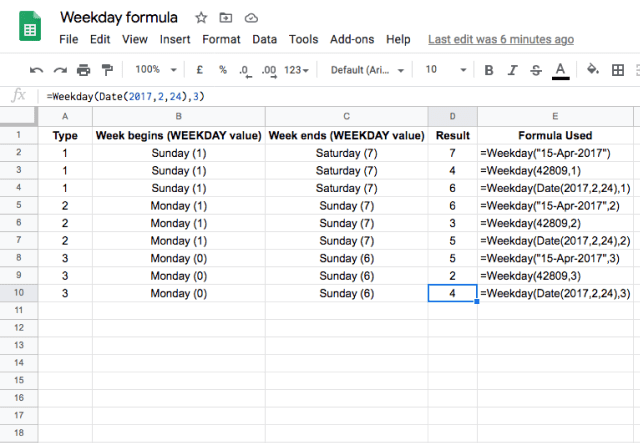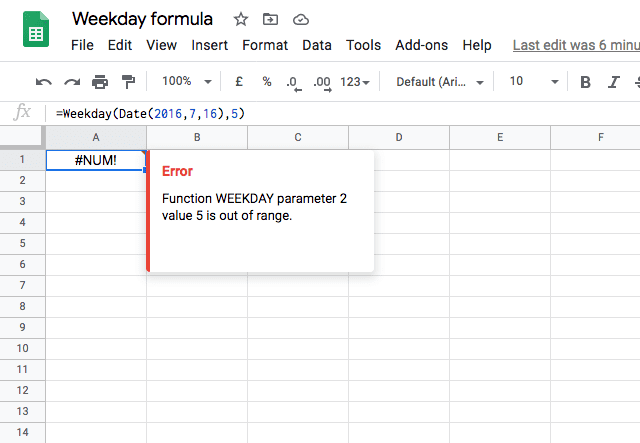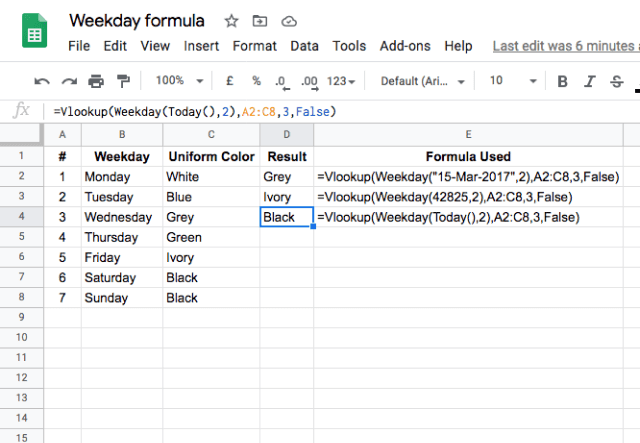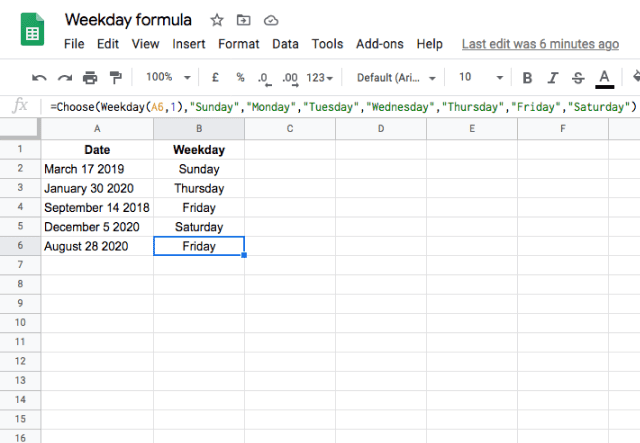# How to use the WEEKDAY function in Google SheetsThere will be instances where we may need to know the day of the week, for a given date. In Google Sheets, the WEEKDAY function can help us with this.

## Syntax

=WEEKDAY(date, [type])
• date – is the input date that the function takes into determining the day of the week. This can be a reference to a cell that holds a date value, or a formula that returns a date or even a number that the Google Sheets can evaluate to a date.
• type – this is an optional indicator that lets the function know where to begin the week from. Valid values that we can key in for this parameter are 1, 2 and 3.
• 1 is the value by default. In this case, the week starts on Sunday and ends on Saturday. Therefore, value for Sunday is 1 and Saturday is 7.
• If we keyed in 2 for this parameter, the week begins on Monday and ends on Sunday. Hence, value for Monday is 1 and Sunday is 7.
• Similarly, if this parameter is 3, the week begins on Monday and ends on Sunday. But the difference here is that the value for Monday is 0 and Sunday is 6.

## How to use WEEKDAY function

I’ll dive into a few examples to provide a better understanding of the function’s working behaviour. Please consider the snapshot below.You will notice I have illustrated all types of WEEKDAY function types (as shown in column A). For the purpose of clarity, I indicated against each function type, the starting day, ending day and their corresponding WEEKDAY values in columns B and C.

As per the given input type, and the day the input date evaluates to, the function accordingly returns the output (column D). The first three example cases are of type 1 and returned the values 7,4 and 6, which correspond to Saturday, Wednesday, and Friday respectively. The next three cases are of type 2 and returned 6, 3 and 5 for the same dates used in the first three cases. Similarly, the final three cases returned are of type 3. Therefore the results are 5, 2 and 4.

### WEEKDAY out of range

What if you enter a different type other than that mentioned above? You can be sure to see an error, of course! I’ll try that here.You can further extend the use of the WEEKDAY function by using the value it returns as the input to another function. For instance, here’s an example of an employee who needs to wear a different colored uniform each day according to the data shown below.### WEEKDAY and VLOOKUP

The WEEKDAY function is used to determine the day of the week for a date, and then the VLOOKUP function is used to match the weekday with the corresponding uniform color. This is achieved with the formula =Vlookup(Weekday(Today(),2),A2:C8,3,False).

### WEEKDAY and CHOOSE

What if you want to generate the day of the week for a list of dates? There is a simple way to do that using the WEEKDAY function in combination with the CHOOSE function. In the example below, this is done by typing =Choose(Weekday(A6,1),”Sunday”,”Monday”,”Tuesday”,”Wednesday”,”Thursday”,”Friday”,”Saturday”).Here, the WEEKDAY function is the input to the CHOOSE function, which takes the number generated by the WEEKDAY function and uses it to select the appropriate weekday choice.

Make sure to get yourself known with the TODAY function as well in the following blog posts: How to use the TODAY function in Google Sheets and How to use the WEEKNUM formula in Google Sheets.

Editor’s note: This is a revised version of a previous post that has been updated for accuracy and comprehensiveness.